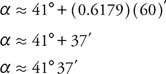## Tables of Trigonometric Functions

Calculators and tables are used to determine values of trigonometric functions. Most scientific calculators have function buttons to find the sine, cosine, and tangent of angles. The size of the angle is entered in degree or radian measure, depending on the setting of the calculator. Degree measure will be used here unless specifically stated otherwise. When solving problems using trigonometric functions, either the angle is known and the value of the trigonometric function must be found, or the value of the trigonometric function is known and the angle must be found. These two processes are inverses of each other. Inverse notations are used to express the angle in terms of the value of the trigonometric function. The expression sin θ = 0.4295 can be written as θ = Sin −10.4295 or θ = Arcsin0.4295 and these two equations are both read as “theta equals Arcsin 0.4295.” Sometimes the expression “inverse sine of 0.4295” is used. Some calculators have a button marked “arc,” which is pressed prior to the function key to express “arc” functions. Arc functions are used to find the measure of the angle if the value of the trigonometric function is known. If tables are used instead of a calculator, the same table is used for either process. Note: The use of calculators or tables gives only approximate answers. Even so, an equal (=) sign is sometimes used instead of an approximate (≈ or ≅) sign.

Example 1: What is the sine of 48°?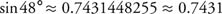Example 2:
What angle has a cosine of 0.3912?Although a calculator can find trigonometric functions of fractional angle measure with ease, this may not be true if you must use a table to look up the values. Tables cannot list all angles. Therefore, approximation must be used to find values between those listed in the table. This method is known as linear interpolation. The assumption is made that differences in function values are directly proportional to the differences of the measures of the angles over small intervals. This is not really true, but yields a better answer than just using the closest value in the table. This method is illustrated in the following examples.

Example 3 : Using linear interpolation, find tan 28.43° given that tan 28.40° = 0.5407 and tan 28.50° = 0.5430.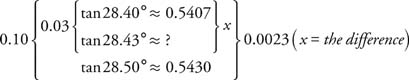Set up a proportion using the variable x.Because x is the difference between tan 28.40° and tan 28.43°,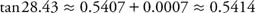Example 4: Find the first quadrant angle α where cos α ≈ 0.2622, given that cos 74° ≈ 0.275 and cost 75° ≈ 0.2588.Set up a proportion using the variable x.Therefore, α ≈ 74.0° + 0.8° ≈ 74.8°

An interesting approximation technique exists for finding the sine and tangent of angles that are less than 0.4 radians (approximately 23°). The sine and tangent of angles less than 0.4 radians are approximately equal to the angle measure. For example, using radian measure, sin0.15 ≈ 0.149 and tan 0.15 ≈ 0.151.

Example 5: Find θ in Figure without using trigonometry tables or a calculator to find the value of any trigonometric functions.Figure 1
Drawing for Example 5.

Because sin θ = 5/23 ≈ 0.21739, the size of the angle can be approximated as 0.217 radians, which is approximately 12.46°. In reality, the answer is closer to 0.219 radians, or 12.56°—quite close for an approximation. If the Pythagorean theorem is used to find the third side of the triangle, the process could also be used on the tangent.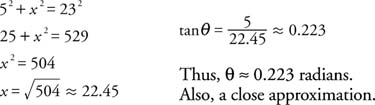Example 6: Find the measure of an acute angle α accurate to the nearest minute if tan α = 0.8884.Using a calculator